Next: Binomial Approx. to Hypergeo.; Up: No Title Previous: The hypergeometric distribution:

# The Binomial Approximation to the Hypergeometric

Suppose we still have the population of size N with M units labelled as success'' and N-M labelled as failure,'' but now we take a sample of size n is drawn with replacement. Then, with each draw, the units remaining to be drawn look the same: still M successes'' and N-M failures.'' Thus, the probability of drawing a success'' on each single draw isand this doesn't change. When we were drawing without replacement, the proportions of successes would change, depending on the result of previous draws. For example, if we were to obtain a success'' on the first draw, then the proportion of successes'' for the second draw would be (M-1)/(N-1), whereas if we were to obtain a failure'' on the first draw the proportion of successes for the second draw would be M/(N-1).

The random variable Y is defined as the number of successes'' in the sample, when we are drawing with replacement. Then Y is a binomial random variable: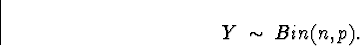The probability mass function for Y is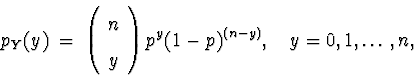and otherwise.

Proposition: If the population size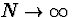in such a way that the proportion of successes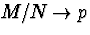,and n is held constant, then the hypergeometric probability mass function approaches the binomial probability mass function: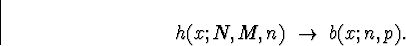In practice, this means that we can approximate the hypergeometric probabilities with binomial probabilities, provided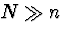.As a rule of thumb, if the population size is more than 20 times the sample size (N > 20 n), then we may use binomial probabilities in place of hypergeometric probabilities.

We next illustrate this approximation in some examples.Next: Binomial Approx. to Hypergeo.; Up: No Title Previous: The hypergeometric distribution:
Dennis Cox
2/12/2001